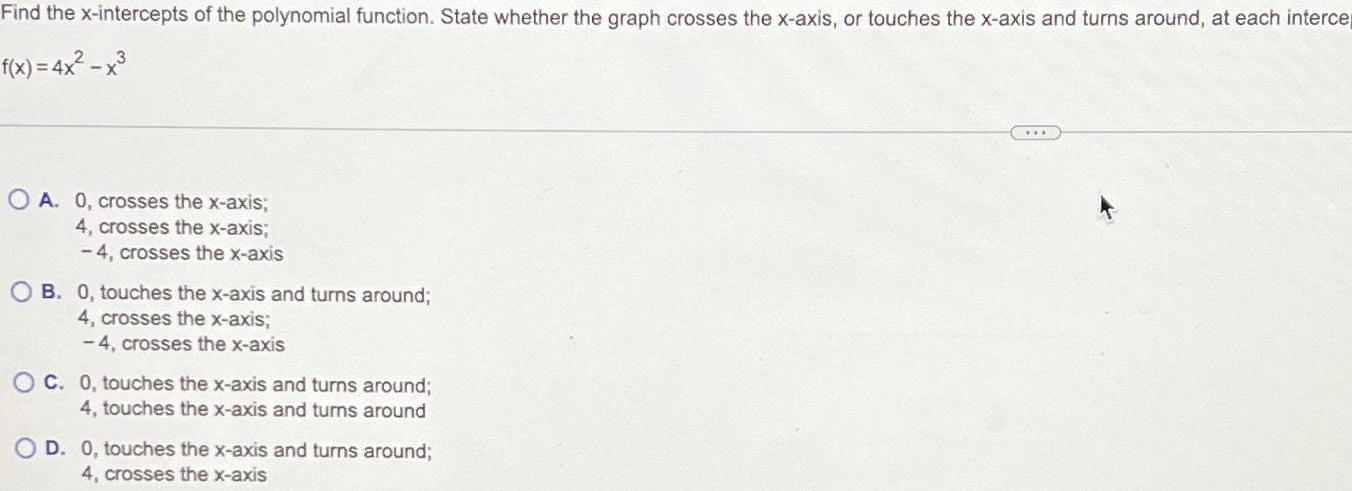Question:

# Find the x intercepts of the polynomial function State

Last updated: 11/15/2023Find the x intercepts of the polynomial function State whether the graph crosses the x axis or touches the x axis and turns around at each interce f x 4x x OA 0 crosses the x axis 4 crosses the x axis 4 crosses the x axis OB 0 touches the x axis and turns around 4 crosses the x axis 4 crosses the x axis OC 0 touches the x axis and turns around 4 touches the x axis and turns around D O touches the x axis and turns around 4 crosses the x axis